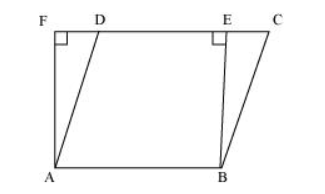# Parallelogram ABCD and rectangle ABEF are on the same base AB and have equal areas.`
Question. Parallelogram ABCD and rectangle ABEF are on the same base AB and have equal areas. Show that the perimeter of the parallelogram is greater than that of the rectangle.

Solution:

As the parallelogram and the rectangle have the same base and equal area, therefore, these will also lie between the same parallels.

Consider the parallelogram ABCD and rectangle ABEF as follows.Here, it can be observed that parallelogram ABCD and rectangle ABEF are between the same parallels AB and CF.

We know that opposite sides of a parallelogram or a rectangle are of equal lengths. Therefore,

$A B=E F$ (For rectangle)

$A B=C D$ (For parallelogram)

$\therefore C D=E F$

$\Rightarrow A B+C D=A B+E F$...(1)

Of all the line segments that can be drawn to a given line from a point not lying on it, the perpendicular line segment is the shortest.

$\therefore \mathrm{AF}<\mathrm{AD}$

And similarly, BE < BC

AF + BE < AD + BC ... (2)

From equations (1) and (2), we obtain

AB + EF + AF + BE < AD + BC + AB + CD

Perimeter of rectangle ABEF < Perimeter of parallelogram ABCD